# Selina Solutions Concise Mathematics Class 6 Chapter 10 Sets Exercise 10(D)

Selina Solutions Concise Mathematics Class 6 Chapter 10 Sets Exercise 10(D) has accurate Solutions for the textbook questions, as per the current syllabus prescribed by the ICSE. Students, who aspire to understand the concepts easily, are suggested to solve the problems of textbooks. Selina Solutions increases students’ confidence in solving complex problems with ease, that would appear in exams. Practising Selina Solutions on a regular basis helps them to develop better academic performance. The Solutions PDF is available online and offline, whenever necessary, with a free download option. Selina Solutions Concise Mathematics Class 6 Chapter 10 Sets Exercise 10(D) PDF provided from the links below for free download

## Selina Solutions Concise Mathematics Class 6 Chapter 10: Sets Exercise 10(D) Download PDF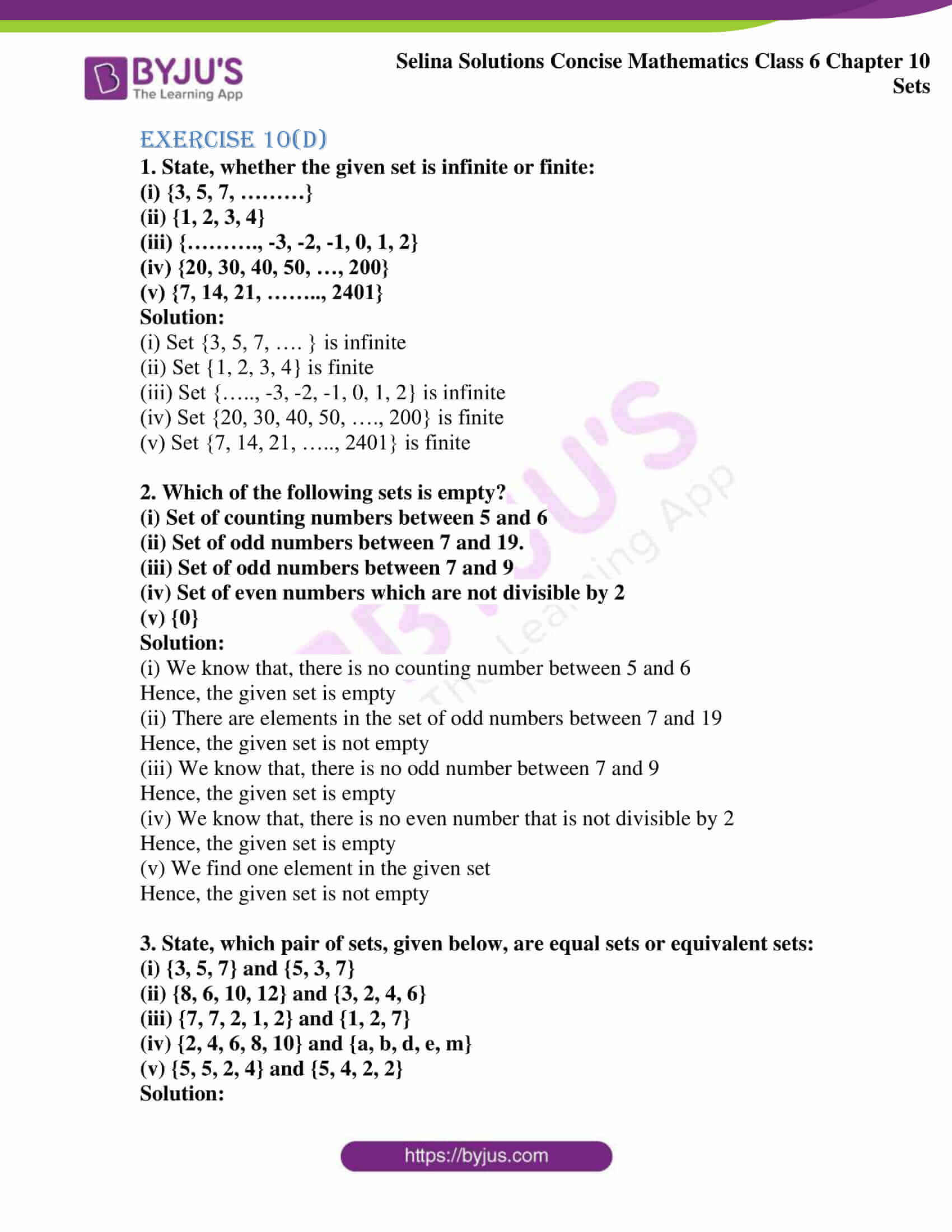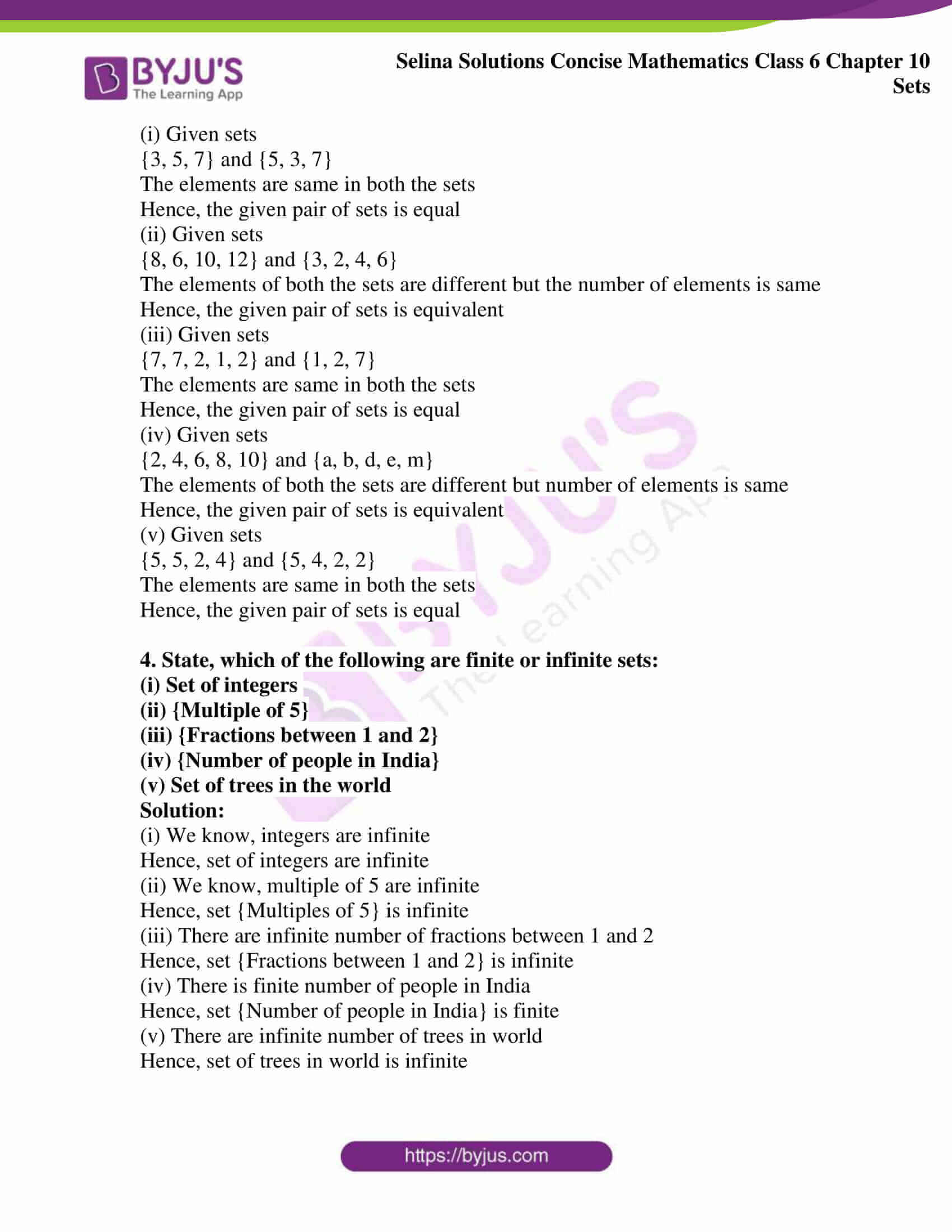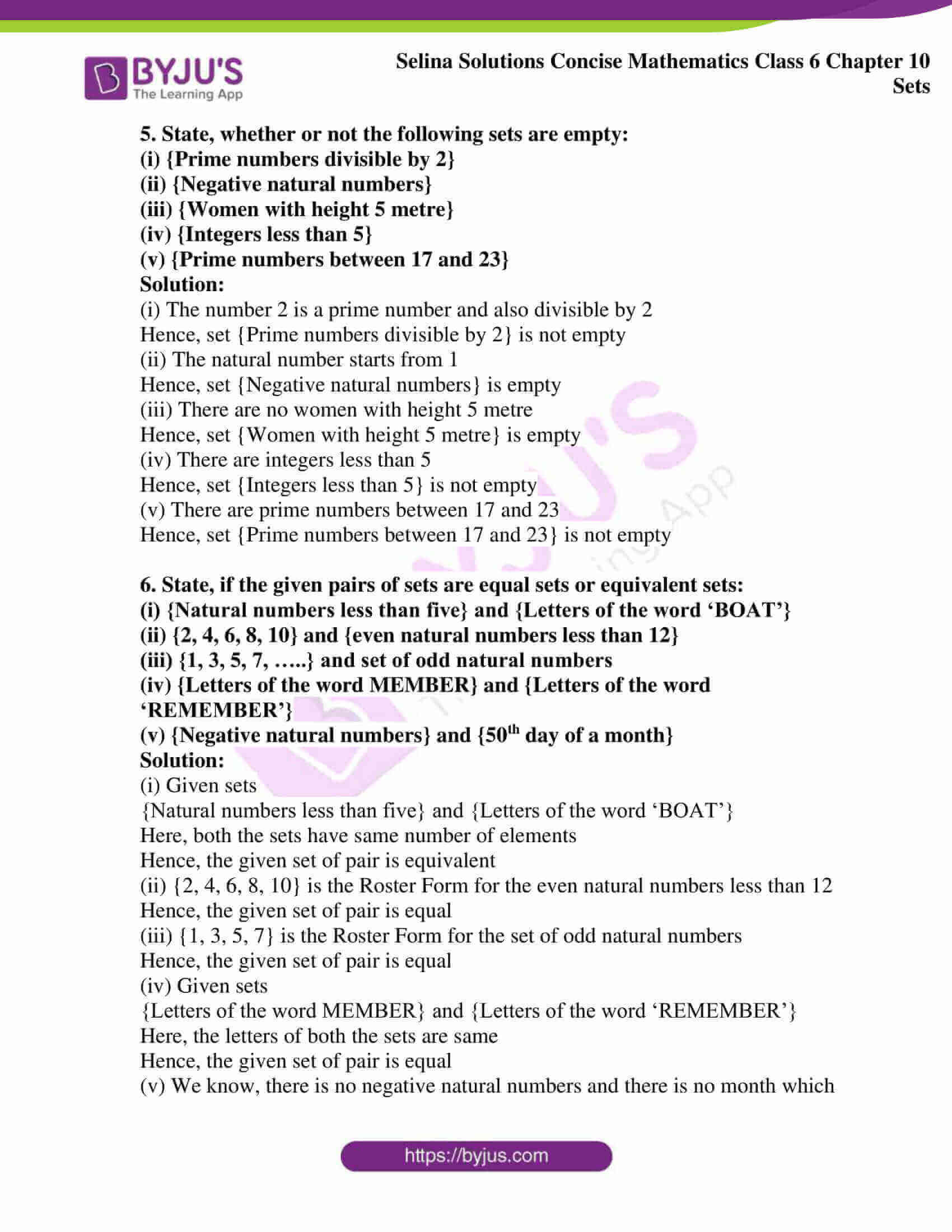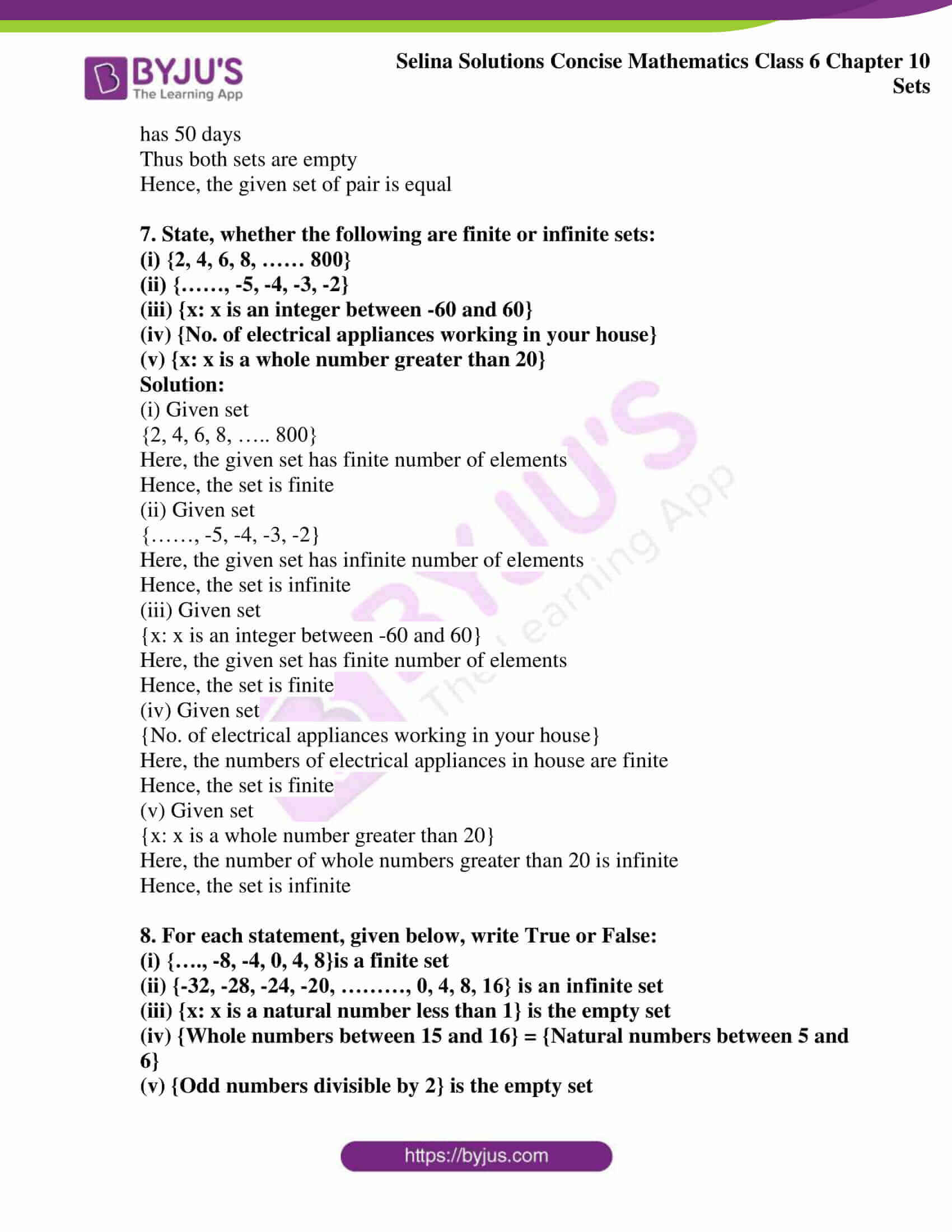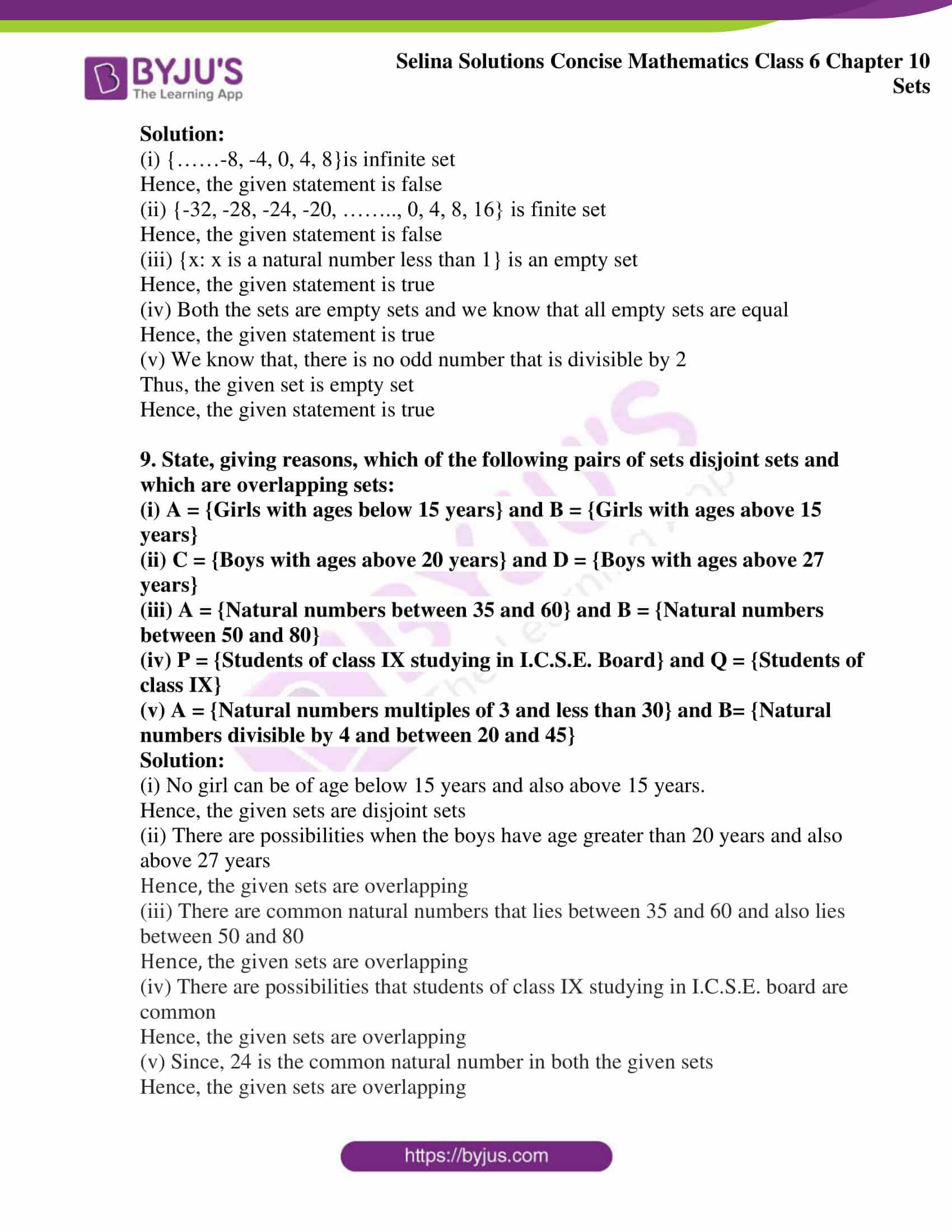### Access other exercise of Selina Solutions Concise Mathematics Class 6 Chapter 10: Sets

Exercise 10(A) Solutions

Exercise 10(B) Solutions

Exercise 10(C) Solutions

Exercise 10(E) Solutions

### Access Selina Solutions Concise Mathematics Class 6 Chapter 10: Sets Exercise 10(D)

#### Exercise 10(D)

1. State, whether the given set is infinite or finite:

(i) {3, 5, 7, ………}

(ii) {1, 2, 3, 4}

(iii) {………., -3, -2, -1, 0, 1, 2}

(iv) {20, 30, 40, 50, …, 200}

(v) {7, 14, 21, …….., 2401}

Solution:

(i) Set {3, 5, 7, …. } is infinite

(ii) Set {1, 2, 3, 4} is finite

(iii) Set {….., -3, -2, -1, 0, 1, 2} is infinite

(iv) Set {20, 30, 40, 50, …., 200} is finite

(v) Set {7, 14, 21, ….., 2401} is finite

2. Which of the following sets is empty?

(i) Set of counting numbers between 5 and 6

(ii) Set of odd numbers between 7 and 19.

(iii) Set of odd numbers between 7 and 9

(iv) Set of even numbers which are not divisible by 2

(v) {0}

Solution:

(i) We know that, there is no counting number between 5 and 6

Hence, the given set is empty

(ii) There are elements in the set of odd numbers between 7 and 19

Hence, the given set is not empty

(iii) We know that, there is no odd number between 7 and 9

Hence, the given set is empty

(iv) We know that, there is no even number that is not divisible by 2

Hence, the given set is empty

(v) We find one element in the given set

Hence, the given set is not empty

3. State, which pair of sets, given below, are equal sets or equivalent sets:

(i) {3, 5, 7} and {5, 3, 7}

(ii) {8, 6, 10, 12} and {3, 2, 4, 6}

(iii) {7, 7, 2, 1, 2} and {1, 2, 7}

(iv) {2, 4, 6, 8, 10} and {a, b, d, e, m}

(v) {5, 5, 2, 4} and {5, 4, 2, 2}

Solution:

(i) Given sets

{3, 5, 7} and {5, 3, 7}

The elements are same in both the sets

Hence, the given pair of sets is equal

(ii) Given sets

{8, 6, 10, 12} and {3, 2, 4, 6}

The elements of both the sets are different but the number of elements is same

Hence, the given pair of sets is equivalent

(iii) Given sets

{7, 7, 2, 1, 2} and {1, 2, 7}

The elements are same in both the sets

Hence, the given pair of sets is equal

(iv) Given sets

{2, 4, 6, 8, 10} and {a, b, d, e, m}

The elements of both the sets are different but number of elements is same

Hence, the given pair of sets is equivalent

(v) Given sets

{5, 5, 2, 4} and {5, 4, 2, 2}

The elements are same in both the sets

Hence, the given pair of sets is equal

4. State, which of the following are finite or infinite sets:

(i) Set of integers

(ii) {Multiple of 5}

(iii) {Fractions between 1 and 2}

(iv) {Number of people in India}

(v) Set of trees in the world

Solution:

(i) We know, integers are infinite

Hence, set of integers are infinite

(ii) We know, multiple of 5 are infinite

Hence, set {Multiples of 5} is infinite

(iii) There are infinite number of fractions between 1 and 2

Hence, set {Fractions between 1 and 2} is infinite

(iv) There is finite number of people in India

Hence, set {Number of people in India} is finite

(v) There are infinite number of trees in world

Hence, set of trees in world is infinite

5. State, whether or not the following sets are empty:

(i) {Prime numbers divisible by 2}

(ii) {Negative natural numbers}

(iii) {Women with height 5 metre}

(iv) {Integers less than 5}

(v) {Prime numbers between 17 and 23}

Solution:

(i) The number 2 is a prime number and also divisible by 2

Hence, set {Prime numbers divisible by 2} is not empty

(ii) The natural number starts from 1

Hence, set {Negative natural numbers} is empty

(iii) There are no women with height 5 metre

Hence, set {Women with height 5 metre} is empty

(iv) There are integers less than 5

Hence, set {Integers less than 5} is not empty

(v) There are prime numbers between 17 and 23

Hence, set {Prime numbers between 17 and 23} is not empty

6. State, if the given pairs of sets are equal sets or equivalent sets:

(i) {Natural numbers less than five} and {Letters of the word ‘BOAT’}

(ii) {2, 4, 6, 8, 10} and {even natural numbers less than 12}

(iii) {1, 3, 5, 7, …..} and set of odd natural numbers

(iv) {Letters of the word MEMBER} and {Letters of the word ‘REMEMBER’}

(v) {Negative natural numbers} and {50th day of a month}

Solution:

(i) Given sets

{Natural numbers less than five} and {Letters of the word ‘BOAT’}

Here, both the sets have same number of elements

Hence, the given set of pair is equivalent

(ii) {2, 4, 6, 8, 10} is the Roster Form for the even natural numbers less than 12

Hence, the given set of pair is equal

(iii) {1, 3, 5, 7} is the Roster Form for the set of odd natural numbers

Hence, the given set of pair is equal

(iv) Given sets

{Letters of the word MEMBER} and {Letters of the word ‘REMEMBER’}

Here, the letters of both the sets are same

Hence, the given set of pair is equal

(v) We know, there is no negative natural numbers and there is no month which has 50 days

Thus both sets are empty

Hence, the given set of pair is equal

7. State, whether the following are finite or infinite sets:

(i) {2, 4, 6, 8, …… 800}

(ii) {……, -5, -4, -3, -2}

(iii) {x: x is an integer between -60 and 60}

(iv) {No. of electrical appliances working in your house}

(v) {x: x is a whole number greater than 20}

Solution:

(i) Given set

{2, 4, 6, 8, ….. 800}

Here, the given set has finite number of elements

Hence, the set is finite

(ii) Given set

{……, -5, -4, -3, -2}

Here, the given set has infinite number of elements

Hence, the set is infinite

(iii) Given set

{x: x is an integer between -60 and 60}

Here, the given set has finite number of elements

Hence, the set is finite

(iv) Given set

{No. of electrical appliances working in your house}

Here, the numbers of electrical appliances in house are finite

Hence, the set is finite

(v) Given set

{x: x is a whole number greater than 20}

Here, the number of whole numbers greater than 20 is infinite

Hence, the set is infinite

8. For each statement, given below, write True or False:

(i) {…., -8, -4, 0, 4, 8}is a finite set

(ii) {-32, -28, -24, -20, ………, 0, 4, 8, 16} is an infinite set

(iii) {x: x is a natural number less than 1} is the empty set

(iv) {Whole numbers between 15 and 16} = {Natural numbers between 5 and 6}

(v) {Odd numbers divisible by 2} is the empty set

Solution:

(i) {……-8, -4, 0, 4, 8}is infinite set

Hence, the given statement is false

(ii) {-32, -28, -24, -20, …….., 0, 4, 8, 16} is finite set

Hence, the given statement is false

(iii) {x: x is a natural number less than 1} is an empty set

Hence, the given statement is true

(iv) Both the sets are empty sets and we know that all empty sets are equal

Hence, the given statement is true

(v) We know that, there is no odd number that is divisible by 2

Thus, the given set is empty set

Hence, the given statement is true

9. State, giving reasons, which of the following pairs of sets disjoint sets and which are overlapping sets:

(i) A = {Girls with ages below 15 years} and B = {Girls with ages above 15 years}

(ii) C = {Boys with ages above 20 years} and D = {Boys with ages above 27 years}

(iii) A = {Natural numbers between 35 and 60} and B = {Natural numbers between 50 and 80}

(iv) P = {Students of class IX studying in I.C.S.E. Board} and Q = {Students of class IX}

(v) A = {Natural numbers multiples of 3 and less than 30} and B= {Natural numbers divisible by 4 and between 20 and 45}

Solution:

(i) No girl can be of age below 15 years and also above 15 years.

Hence, the given sets are disjoint sets

(ii) There are possibilities when the boys have age greater than 20 years and also above 27 years

Hence, the given sets are overlapping

(iii) There are common natural numbers that lies between 35 and 60 and also lies between 50 and 80

Hence, the given sets are overlapping

(iv) There are possibilities that students of class IX studying in I.C.S.E. board are common

Hence, the given sets are overlapping

(v) Since, 24 is the common natural number in both the given sets

Hence, the given sets are overlapping